# AP Board 8th Class Maths Solutions Chapter 6 Square Roots and Cube Roots Ex 6.3

AP State Syllabus AP Board 8th Class Maths Solutions Chapter 6 Square Roots and Cube Roots Ex 6.3 Textbook Questions and Answers.

## AP State Syllabus 8th Class Maths Solutions 6th Lesson Square Roots and Cube Roots Exercise 6.3Question 1.
Find the square roots of the following numbers by division method.
(i) 1089
(ii) 2304
(iii) 7744
(iv) 6084
(v) 9025
Solution:
(i) 1089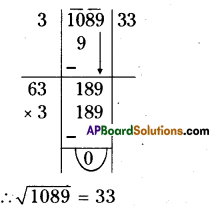(ii) 2304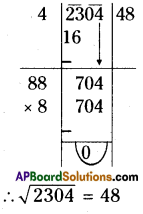(iii) 7744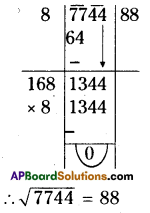(iv) 6084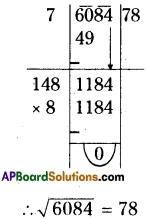(v) 9025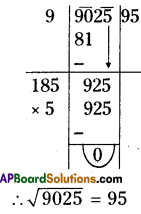Question 2.
Find the square roots of the following decimal numbers.
(i) 2.56
(ii) 18.49
(iii) 68.89
(iv) 84.64
Solution:
(i) 2.56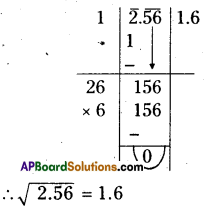(ii) 18.49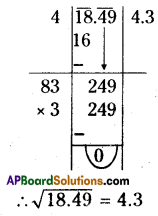(iii) 68.89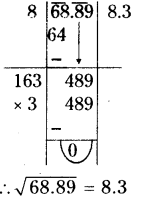(iv) 84.64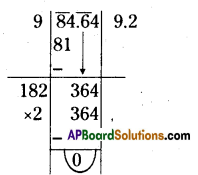Question 3.
Find the least number that is to be subtracted from 4000 to make it perfect square
Solution:
Square root of 4000 by
Division Method: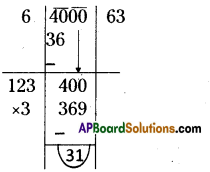∴ The least number 31 should be subtracted from 4000 we will get a perfect square number4
∴ 4000 – 31 = 3969
= $$\sqrt{3969}=\sqrt{63 \times 63}$$ = 63Question 4.
Find the length of the side of a square whose area is 4489 sq.cm.
Solution:
Area of a square (A) = 4489 sq.cms
A = s2
s2 = 4489
s = $$\sqrt{4489}=\sqrt{67 \times 67}$$ = 67cms.
∴ The side of a square (s) = 67cms.

Question 5.
A gardener wishes to plant 8289 plants in the form of a square and found that there were 8 plants left. How many plants were planted in each row?
Solution:
No. of plants are planted = 8289 If 8289 plants are planted in a square shape, 8 plants are left.
Then remaining plants = 8289 – 8 = 8281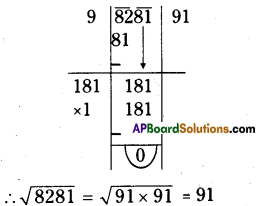∴ No. of plants for each row = 91
∴ 8281 plants are planted in a square shape then no. of plants are planted for each row = 91Question 6.
Find the least perfect square with four digits.
Solution:
The smallest number of 4 digits = 1000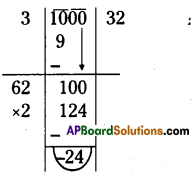∴ 24 should be added tö 1000 then 1000 + 24 = 1024
∴ The smallest 4 digited perfect square number is 1024.
[∵ $$\sqrt{1024}$$ = 32]Question 7.
Find the least number which must be added to 6412 to make it a perfect square?
Solution: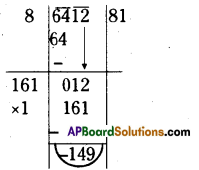∴ The least number 149 should be added to 6412 then we will get a perfect square number.
∴ 6412 + 149 = 6561
∴ $$\sqrt{6561}=\sqrt{81 \times 81}$$ = 81

Question 8.
Estimate the value of the following numbers to the nearest whole number
(i) $$\sqrt{97}$$
(ii) $$\sqrt{250}$$
(iii) $$\sqrt{780}$$
Solution:
ï) $$\sqrt{97}$$ , 97 lie between the perfect
square numbers 81 and 100.
∴ 81 <97< 100
92 < 97 < 102
=9 < $$\sqrt{97}$$ < 10
∴ $$\sqrt{97}$$ Is nearest to 10.
[∵ 97 is nearest to 100]

(ii) $$\sqrt{250}$$, 250 lie between the perfect square numbers 225 and 256.
∴ 225 < 250 < 256
152 < 250 < 162
= 15 < $$\sqrt{250}$$ <16
∴ $$\sqrt{250}$$ is nearest to 16.
[ ∵ 250 is nearest to 256]ii) $$\sqrt{780}$$, 780 lie between the perfect
square numbers 729 and 784.
∴ 729 < 780 < 784
272 < 780 < 282
= 27< $$\sqrt{780}$$ <28
∴ $$\sqrt{780}$$ is nearest to 28.
[ ∵ 780 is nearest to 784]